# Test: Determinants (CBSE Level) - 1

## 25 Questions MCQ Test Mathematics (Maths) Class 12 | Test: Determinants (CBSE Level) - 1

Description
This mock test of Test: Determinants (CBSE Level) - 1 for JEE helps you for every JEE entrance exam. This contains 25 Multiple Choice Questions for JEE Test: Determinants (CBSE Level) - 1 (mcq) to study with solutions a complete question bank. The solved questions answers in this Test: Determinants (CBSE Level) - 1 quiz give you a good mix of easy questions and tough questions. JEE students definitely take this Test: Determinants (CBSE Level) - 1 exercise for a better result in the exam. You can find other Test: Determinants (CBSE Level) - 1 extra questions, long questions & short questions for JEE on EduRev as well by searching above.
QUESTION: 1

### Let a =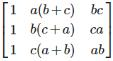, then Det. A is

Solution: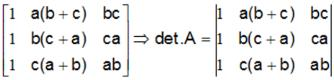Apply C2 → C2 + C3,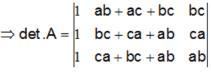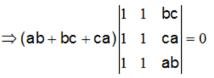QUESTION: 2

###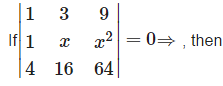Solution: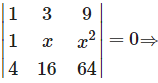because , the value of the determinant is zero only when , the two of its rows are identical., Which is possible only when Either x = 3 or x = 4 .

QUESTION: 3

###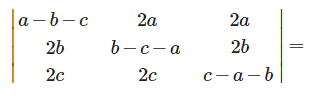Solution: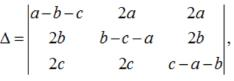Apply , R1 → R1+R2+R3,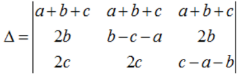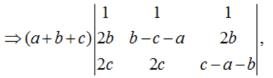Apply , C3→ C- C1, C2C2 - C1,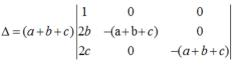=(a+b+c)3

QUESTION: 4

If A and B are invertible matrices of order 3 , then det (adj A) =

Solution:

Let A be a non singular square matrix of order n . then , |adj.A| = A−1

QUESTION: 5

If A and B matrices are of same order and A + B = B + A, this law is known as

Solution:

Commutative law, in mathematics, either of two laws relating to number operations of addition and multiplication, stated symbolically: a + b = b + a and ab = ba

QUESTION: 6

If A’ is the transpose of a square matrix A , then

Solution:

The determinant of a matrix A and its transpose always same.

QUESTION: 7

The value of the determinant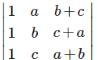is

Solution:, Apply , C2 → C2 + C3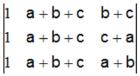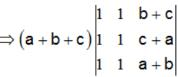= 0, (∵ C1 = C2)

QUESTION: 8

The roots of the equation det.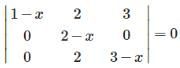are

Solution: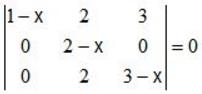⇒ (1-x)(2-x)(3-x) = 0 ⇒x = 1,2,3

QUESTION: 9

If A is a square matrix of order 2 , then det (adj A) = x

Solution:

Let A be a square matrix of order 2 . then ,|adj.A| = |A|

QUESTION: 10

If A is a symmetric matrix, then At =

Solution:
QUESTION: 11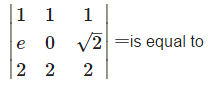Solution: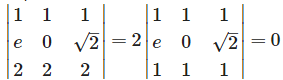, because , row 1 and row 3 are identical.

QUESTION: 12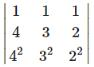is equal to

Solution: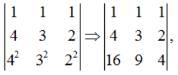Apply , C1→C1 - C3, C2→C2-C3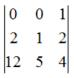= 10 - 12 = -2

QUESTION: 13

If A+B+C = π, then the value of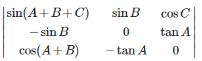Solution: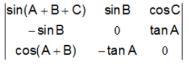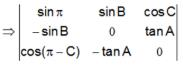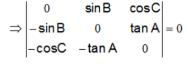QUESTION: 14

If A is a non singular matrix of order 3 , then |adj(A3)| =

Solution:

If A is anon singular matrix of order , then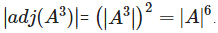QUESTION: 15

If A and B are any 2 × 2 matrices , then det. (A+B) = 0 implies

Solution:

Det.(A+B) ≠ Det.A + Det.B.

QUESTION: 16

If A B be two square matrices such that AB = O, then

Solution:

If A B be two square matrices such that AB = O, then,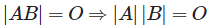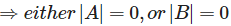QUESTION: 17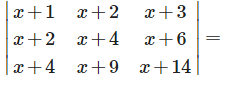Solution: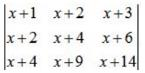Apply , C1 → C1 - C2, C2 → C2 - C3,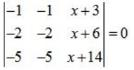Because here row 1 and 2 are identical

QUESTION: 18

If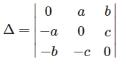, then equals

Solution:

Because , the determinant of a skew symmetric matrix of odd order is always zero and of even order is a non zero perfect square.

QUESTION: 19

If I3 is the identity matrix of order 3 , then 13−1 is

Solution:

Because , the inverse of an identity matrix is an identity matrix.

QUESTION: 20

If A and B are square matrices of same order and A’ denotes the transpose of A , then

Solution:

By the property of transpose of a matrix ,(AB)’ = B’A’.

QUESTION: 21

A square matrix A is invertible iff det A is equal to

Solution:

Only non-singular matrices possess inverse.

QUESTION: 22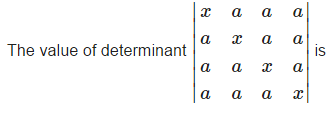Solution: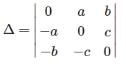Apply, C1→ C1+ C2+C3+C4,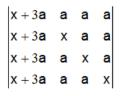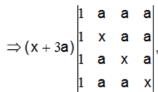Apply, R1 →R1 - R2,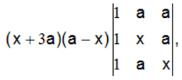Apply, R1 R- R2, R→ R- R3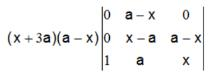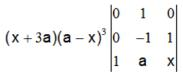=(x+3a) (a -x)3 (1) = (x+3a)(a-x)3

QUESTION: 23

If the entries in a 3 x 3 determinant are either 0 or 1 , then the greatest value of this determinant is :

Solution:

Greatest value = 2

QUESTION: 24

The roots of the equation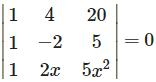are

Solution:

Operate,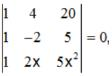Apply R3→R3- R1, R2 R2 -R1,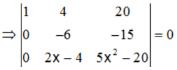⇒ -6(5x2 - 20) +15(2x-4) = 0

⇒ (x- 2)(x+1) = 0⇒x=2, -1

⇒-6(5x2 - 20) + 15(2x-4) = 0

⇒(x-2)(x+1) = 0 ⇒ x=2, -1

QUESTION: 25

In a third order determinant, each element of the first column consists of sum of two terms, each element of the second column consists of sum of three terms and each element of the third column consists of sum of four terms. Then it can be decomposed into n determinants, where n has value

Solution:

N = 2 ×3 × 4 = 24.